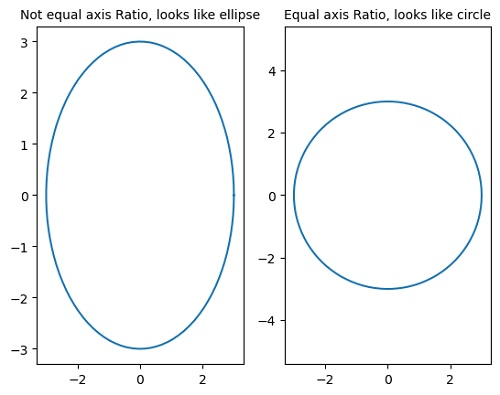# Python | Equal Axis Scale Ratio in Plotting

Here, we are going to learn about the equal axis scale ratio in plotting and its Python implementation.
Submitted by Anuj Singh, on July 31, 2020

In some cases, the axis ratio is important such as, the axis must be on the same scale. Our example illustrates that a circle can be an ellipse if the axis scales are not the same. We have an inbuilt defined function within matplotlib.pyplot library for this operation and the following example shows the illustration.## Python code for equal axis scale ratio in plotting

```import numpy as np
import matplotlib.pyplot as plt

an = np.linspace(0, 2*np.pi, 100)

plt.subplot(121)
plt.plot(3*np.cos(an), 3*np.sin(an))
plt.title('Not equal axis Ratio, looks like ellipse', fontsize=10)

plt.subplot(122)
plt.plot(3*np.cos(an), 3*np.sin(an))
plt.axis('equal')
plt.title('Equal axis Ratio, looks like circle', fontsize=10)
```

Output:

```Output is as figure
```